# Procedure - To illustrate that the Medians of a Triangle Concur at a Point (called the Centroid) Class 9 Notes | EduRev

## Class 9 : Procedure - To illustrate that the Medians of a Triangle Concur at a Point (called the Centroid) Class 9 Notes | EduRev

The document Procedure - To illustrate that the Medians of a Triangle Concur at a Point (called the Centroid) Class 9 Notes | EduRev is a part of the Class 9 Course Mathematics (Maths) Class 9.
All you need of Class 9 at this link: Class 9

As performed in real lab:

Materials required:

Coloured paper, pencil, a pair of scissors, gum.

Procedure:

1. From a sheet of paper, cut out three types of triangle: acute-angled triangle, right-angled triangle and obtuse-angle triangle.

2. For an acute-angled triangle, find the mid-points of the sides by bringing the corresponding two vertices together. Make three folds such that each Joins a vertex to the mid-point of the opposite side. [Fig (a)]

3. Repeat the same activity for a right-angled triangle and an obtuse-angled triangle. [Fig (b) and Fig (c)]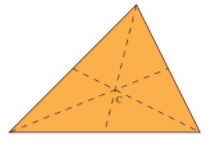Acute-angled(a)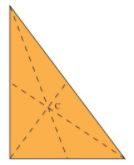Right-angled(b)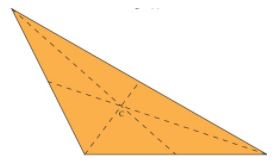Obtuse-angled(c)

As performed in the simulator:

1. Create a triangle ABC by providing three points A, B and C over the workbench.

2. Draw the mid-points of each line segment.

3. Click on each mid-points to draw their respective bisector lines.

4. You can see, Centroid lies inside the triangle for all acute angled, obtuse angled & right angled triangle.

Observations:

• The students observe that the three medians of a triangle concur.

• They also observe that the centroid of an acute, obtuse or right angled triangle always lies inside the triangle.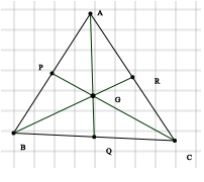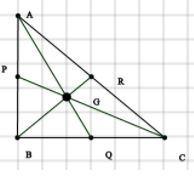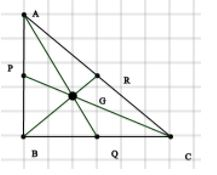Offer running on EduRev: Apply code STAYHOME200 to get INR 200 off on our premium plan EduRev Infinity!

## Mathematics (Maths) Class 9

190 videos|233 docs|82 tests

,

,

,

,

,

,

,

,

,

,

,

,

,

,

,

,

,

,

,

,

,

;